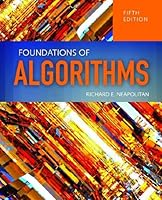# Foundations Of Algorithms, 5th Edition## Book Description

Foundations of , Fifth Edition offers a well-balanced presentation of algorithm design, complexity analysis of , and computational complexity. Ideal for any students with a background in college and discrete structures, the text presents mathematical concepts using standard English and simple notation to maximize and user-friendliness. Concrete examples, appendices reviewing essential mathematical concepts, and a student-focused approach reinforce theoretical explanations and promote learning and retention. C++ and Java pseudocode help students better understand complex algorithms. A chapter on numerical algorithms includes a review of basic number , Euclid's Algorithm for finding the greatest common divisor, a review of modular arithmetic, an algorithm for solving modular linear equations, an algorithm for computing modular powers, and the new polynomial-time algorithm for determining whether a number is prime. The revised and updated Fifth Edition features an all-new chapter on genetic algorithms and genetic programming, including approximate solutions to the traveling salesperson problem, an algorithm for an artificial ant that navigates along a trail of food, and an application to financial trading. With fully updated exercises and examples throughout and improved instructor resources including complete solutions, an Instructor’s Manual and lecture outlines, Foundations of Algorithms is an essential text for undergraduate and graduate courses in the design and analysis of algorithms.

Key features include:

• The only text of its kind with a chapter on genetic algorithms
• Use of C++ and Java pseudocode to help students better understand complex algorithms
• No calculus background required
• Numerous clear and student-friendly examples throughout the text
• Fully updated exercises and examples throughout
• Improved instructor resources, including complete solutions, an Instructor’s Manual, and PowerPoint lecture outlines

Chapter 1 Algorithms: Efficiency, Analysis, and Order
Chapter 2 Divide-and-Conquer
Chapter 3 Dynamic Programming
Chapter 4 The Greedy Approach
Chapter 5 Backtracking
Chapter 6 Branch-and-Bound
Chapter 7 Introduction to Computational Complexity: The Sorting Problem
Chapter 8 More Computational Complexity: The Searching Problem
Chapter 9 Computational Complexity and Intractability: An Introduction to the Theory of NP
Chapter 10 Genetic Algorithms and Genetic Programming
Chapter 11 Number-Theoretic Algorithms
Chapter 12 Introduction to Parallel Algorithms

Appendix A Review of Necessary Mathematics 565 A.1 Notation
Appendix B Solving Recurrence Equations: With Applications to Analysis of Recursive Algorithms
Appendix C Data Structures for Disjoint Sets

## Book Details

• Title: Foundations Of Algorithms, 5th Edition
• Author:
• Length: 676 pages
• Edition: 5
• Language: English
• Publisher:
• Publication Date: 2014-03-05
• ISBN-10: 1284049191
• ISBN-13: 9781284049190